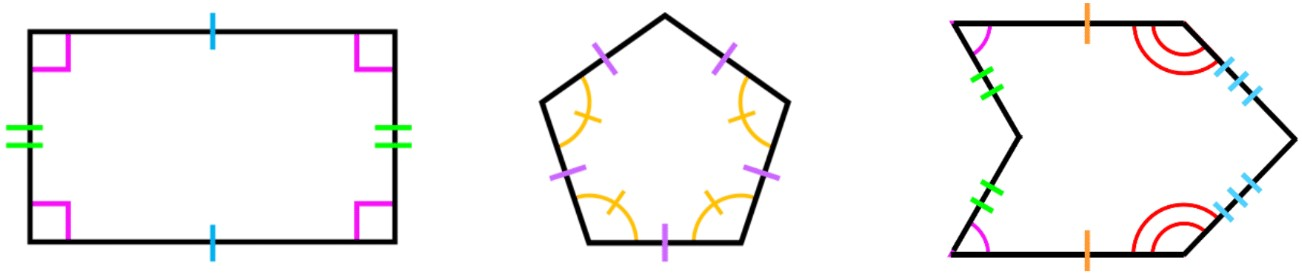# Classifying polygons#### Everything You Need in One Place

Homework problems? Exam preparation? Trying to grasp a concept or just brushing up the basics? Our extensive help & practice library have got you covered.#### Learn and Practice With Ease

Our proven video lessons ease you through problems quickly, and you get tonnes of friendly practice on questions that trip students up on tests and finals.#### Instant and Unlimited Help

Our personalized learning platform enables you to instantly find the exact walkthrough to your specific type of question. Activate unlimited help now!

0/4
##### Intros
###### Lessons
1. Introduction to Classifying Polygons:
2. What are polygons?
3. Names of different polygons based on number of sides (triangles to octagons)
4. Regular or irregular polygons: markings for congruent sides and angles
5. Understanding markings for parallel and perpendicular sides in polygons
0/14
##### Examples
###### Lessons
1. Sides and vertices in polygons
Count the number of sides and vertices in the polygon. Circle the vertices and check mark the sides to help keep track.
1.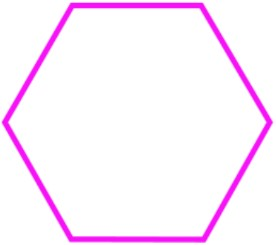2.3.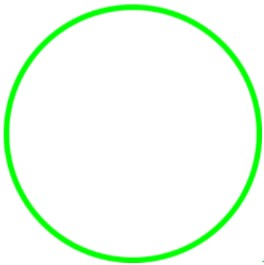2. Classifying polygons by name and regularity
Look at the picture of the shape and write:
• the name of the polygon
• and if it is regular or irregular
1.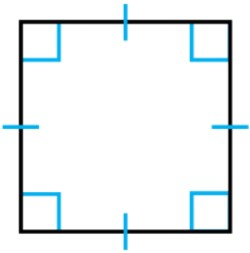2.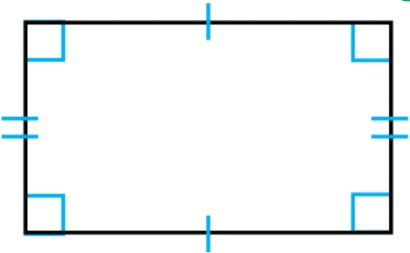3.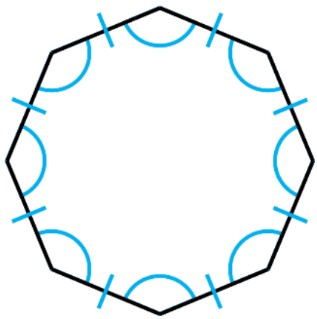4.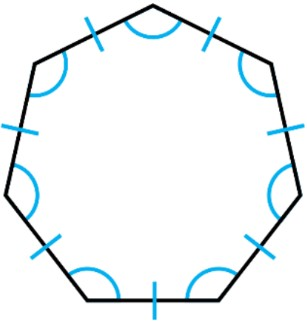5.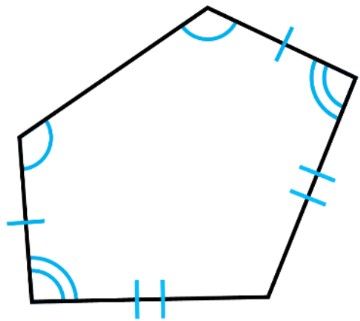3. Congruent sides and angles in polygons
Draw markings for any pairs of equal sides and/or angles
1.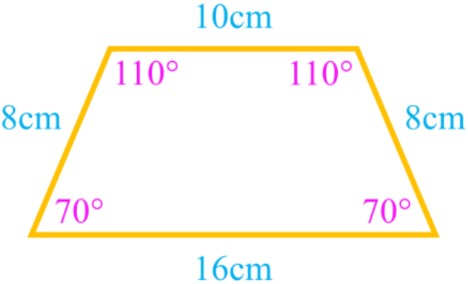2.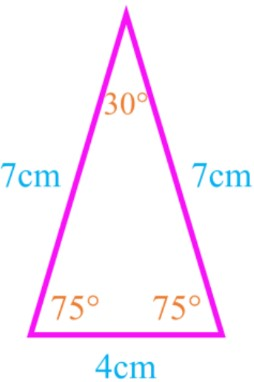3.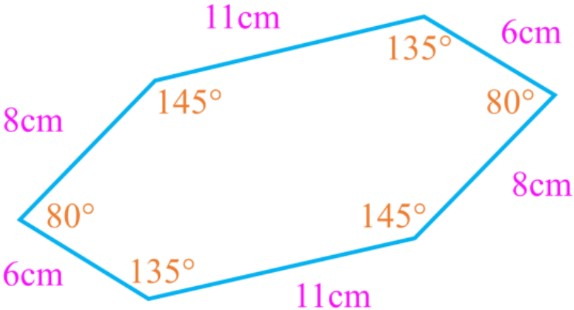4. Parallel sides in polygons
Mark the parallel sides with matching arrows on each pair. How many pairs of sides are parallel?
1.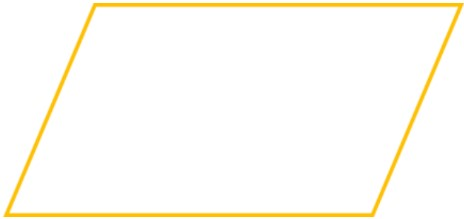2.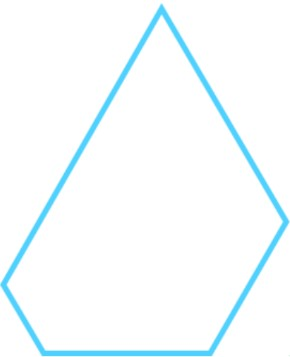3.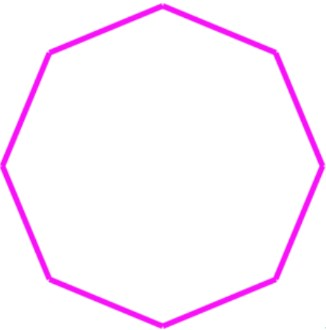0%
##### Practice
###### Topic Notes

In this lesson, we will learn:

• How to name polygons: triangles, quadrilaterals, pentagons, hexagons, heptagons, and octagons
• How to classify regular vs. irregular polygons
• How to read markings on polygons: hashmarks for congruent sides, semicircles for congruent angles, arrows for parallel sides, and squares (right angles) for perpendicular sides

Notes:

• Polygons are 2D shapes with straight sides only. All polygons have sides (straight lines/edges) and vertices (corners/points where sides meet).

• Polygons are named by the number of sides they have: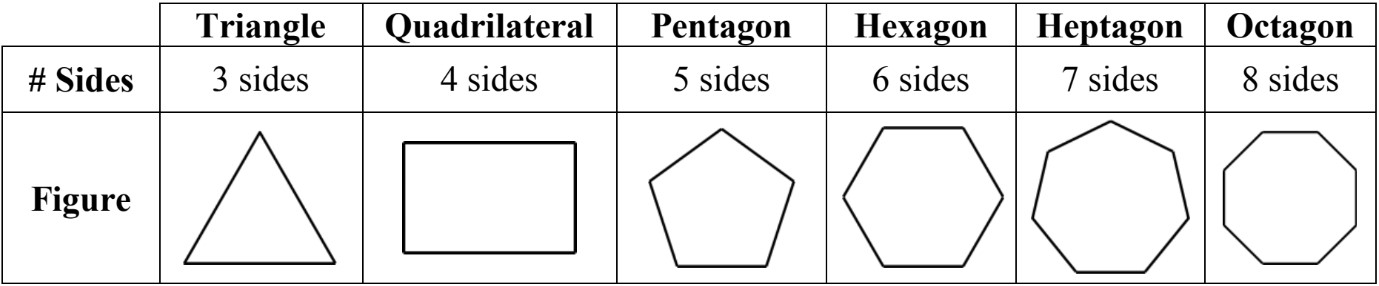• Polygons can be regular or irregular. Regular polygons have congruent sides and angles; all sides and all angles are equal. Irregular polygons do not have same size sides and angles.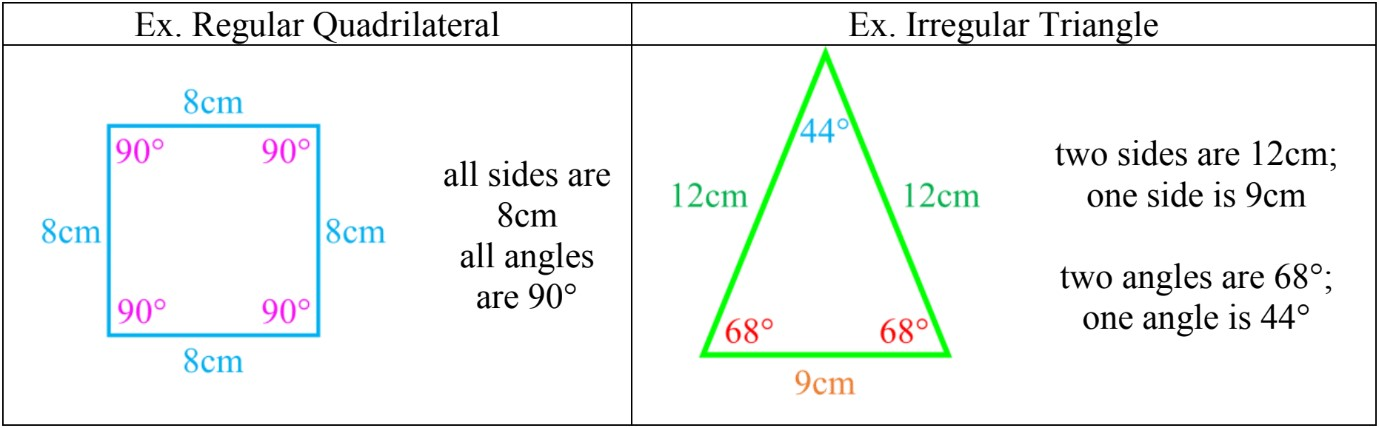• Congruent sides can be shown with hashmarks (matching lines on the sides that are equal)
• Congruent angles can be shown with either semicircles with hashmarks, or with concentric circles